# Non-linear potential

A functiongenerated by a Radon measure,being a point of the Euclidean space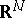,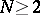, that depends non-linearly on the generating measure.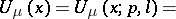(*)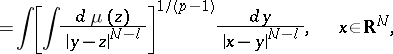whereis the distance betweenand,is a Radon measure with compact support, andandare real numbers,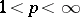,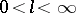.
Forthe non-linear potentials (*) turn into the linear Riesz potentials (cf. Riesz potential), and for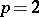and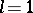into the classical Newton potential. The concepts of capacity and energy have been constructed, and analogues of certain basic theorems of potential theory have been proved for the non-linear potential (*) (see ).